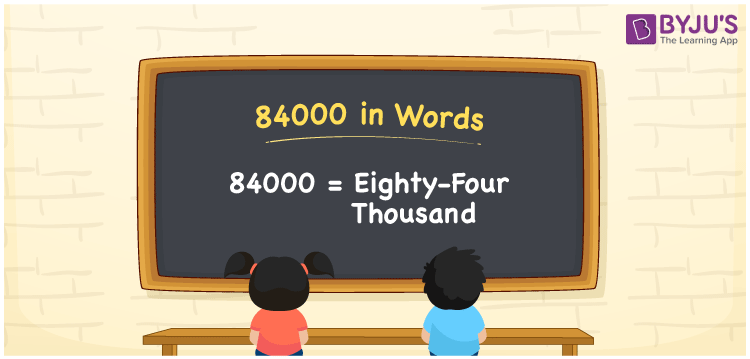# 84000 in words

84000 in words is written as Eighty Four Thousand. In 84000, 8 has a place value of ten thousand and 4 has a place value of thousand. The article on Place Value gives more information. The number 84000 is used in expressions that relate to money, distance, length, count and many more. For example, “Eighty Four Thousand meters is nothing but Eighty Four Kilometers”. This can also be read as “84000 m is the same as 84 km”.

 84000 in words Eighty Four Thousand Eighty Four Thousand in Numbers 84000

## 84000 in English Words## How to Write 84000 in Words?

We can convert 84000 to words using a place value chart. The number 84000 has 5 digits, so let’s make a chart that shows the place value up to 5 digits.

 Ten thousand Thousands Hundreds Tens Ones 8 4 0 0 0

Thus, we can write the expanded form as:

8 × Ten thousand + 4 × Thousand + 0 × Hundred + 0 × Ten + 0 × One

= 8 × 10000 + 4 × 1000 + 0 × 100 + 0 × 10 + 0 × 1

= 84000

= Eighty Four Thousand.

84000 is the natural number that is succeeded by 83999 and preceded by 84001.

84000 in words – Eight Four Thousand.

Is 84000 an odd number? – No.

Is 84000 an even number? – Yes

Is 84000 a perfect square number? – No

Is 84000 a perfect cube number? – No

Is 84000 a prime number? – No

Is 84000 a composite number? – Yes

## Solved Example

1. Write the number 84000 in expanded form

Solution: 8 x 10000 + 4 x 1000 + 0 x 100 + 0 x 10 + 0 x 1

Or Just 8 x 10000 + 4 x 1000

We can write 84000 = 80000 + 4000 + 0 + 0 + 0

= 8 x 10000 + 4 x 1000 + 0 x 100 + 0 x 10 + 0 x 1

## Frequently Asked Questions on 84000 in words

Q1

### How to write 84000 in words?

84000 in words is written as Eighty Four Thousand.
Q2

### State True or False. 84000 is divisible by 4?

True. 84000 is divisible by 4.
Q3

### Is 84000 divisible by 10?

Yes. 84000 is divisible by 10.